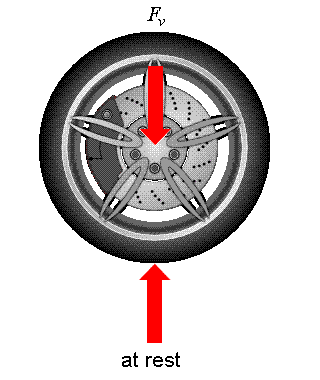Frictional forces of car tires. Translation

So assume a vehicle is undergoing translation in the forward direction on a flat surface, and the power is supplied to the rear wheels.
Lets say the reaction force on the rear wheel is Nr, and on the front it's Nf
U is the coeff. static friction, and theres no slipping.
M is the mass
G = gravity

Ignore air drag.

I know friction on the rear wheel is pointing in the direction of the cars motion. Assuming

Let me break it down into Axes:

Sum in Y(upward):
Nf+Nr = M*G

Sum in X(forward):
M*a = F (where F is frictional force)

Ill skip the moment equation about the center of gravity

My question, is how come i've read that F=U*Nr --- and not F=U*(Nr+Nf)

The front has a normal force, thus -- it should have a frictional force, would it not?
I've read that this force is backwards (kind of makes sense) and is small and is due to the Inertia of the wheel....Can someone explain this to me, or point me to reference that explains this in detail.

Cliff notes:
Why does the frictional only act on the rear wheels?

jack action